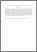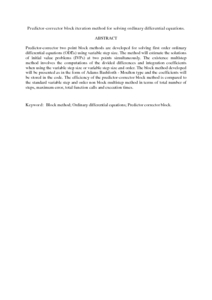# Predictor-corrector block iteration method for solving ordinary differential equations

## Citation

Abdul Majid, Zanariah and Suleiman, Mohamed (2011) Predictor-corrector block iteration method for solving ordinary differential equations. Sains Malaysiana, 40 (6). pp. 659-664. ISSN 0126-6039

## Abstract

Predictor-corrector two point block methods are developed for solving first order ordinary differential equations (ODEs) using variable step size. The method will estimate the solutions of initial value problems (IVPs) at two points simultaneously. The existence multistep method involves the computations of the divided differences and integration coefficients when using the variable step size or variable step size and order. The block method developed will be presented as in the form of Adams Bashforth - Moulton type and the coefficients will be stored in the code. The efficiency of the predictor-corrector block method is compared to the standard variable step and order non block multistep method in terms of total number of steps, maximum error, total function calls and execution times.Preview
PDF (Abstract)
Predictor.pdfView Item